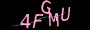×

# 如何使用\$(this).index与\$.each

```\$(function(){
\$(".bao").hide();
\$(".bao").eq(0).show();
\$(".bao").eq(\$(this).index()).show().siblings(".bao").hide()
var a=\$(".bao").eq(\$(this).index()).find('li')
if(a.length<0){
}
});
function moren(){
var moren=\$(".moren").find('li')
if(moren.length==0){
}
}
})```

```\$(function(){
\$(".bao").hide();
\$(".bao").eq(0).show();
\$(".bao").eq(\$(this).index()).show().siblings(".bao").hide()
});
var aaa= \$(".bao ul")
aaa.each(function(){
var b=\$(this).children('li').length
if(b==0){
\$(this).append("<div>我是0个之后增加上去的</div>")
}
})
})```

https://www.jiangweishan.com/article/svg1488297600689.html

### 网友评论文明上网理性发言已有0人参与